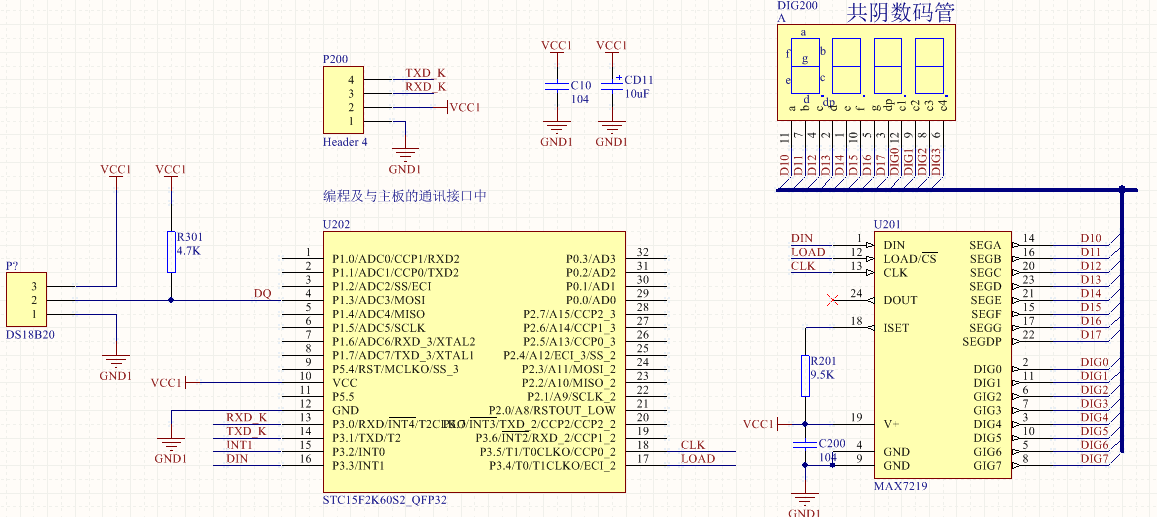Hi 你好，欢迎访问！登录

# 基于STC15单片机及DS18B20的测温实操

###### 2015-10-22 | 实用案例 | caterwang | 3161°c

对于DS18B20，之前在AT89C51上有一次调试经历，后面没再接触过了。这次由于实际需要，又用到了DS18B20。当把旧程序移过去之后，傻眼了，不成功!于是开始了漫长的调试过程!之前有一篇文章也分享过DS18B20的驱动程序了，关于DS18B20的时，大家可以自己查阅手册，只需要记住，这个片子，对时序要求还是高的。这是旨在分享自己真实可用的程序，对于时序部分的控制，为便于大家移植，已经做成固定函数，到时大家把对应的函数改一下就行了。

1. 电路图2.驱动程序--以个人经验，初始化程序很重要```#include <intrins.h>
#include "STC15F2K60S2.H"
#include "DS18B20.H"
#include "max7219.h"
#include "mainapp.h"
void Delay5us()		//@22.1184MHz
{
unsigned char i;

_nop_();
i = 24;
while (--i);
}
void Delay5us_xn(int x)
{
while(x--)
{
Delay5us();
}
}
/**************复位***********************/
unsigned char DS1B20Init(void)
{
unsigned char i;
DQ=1;
_nop_();
DQ=0;
Delay5us_xn(96);			//延时480us
DQ=1;
Delay5us_xn(12);			//延时60us
i=0;
while(DQ)				//在480us内检测DQ是否变为低，如果为低则复位成功，否则复位不成功
{
Delay5us();
i++;
if(i>96)
{
i=255;
break;
}
}
if(i==255)
return 0;                      //初始化失败
while(!DQ);				//等待DS18B20释放总线
return 1;				//初始化成功
}

/**********写一个字节数据到DS18B20***********/
void WriteDat(unsigned char dat)
{
unsigned char i;
for (i=8; i>0; i--) //
{
DQ=1;
_nop_();_nop_();
DQ=0;
Delay5us();
DQ=dat&0x01;      //最低位移出
Delay5us_xn(13);
dat=dat/2;          //右移一位
}
DQ=1;
Delay5us_xn(2);
}
/**********读一个字节数据从DS18B20***********/
{
unsigned char dat,i;
for (i=8;i>0;i--)
{
DQ=1;_nop_();_nop_();
dat>>=1;
DQ=0;             //
Delay5us_xn(1);
DQ=1;
Delay5us_xn(1);
if(DQ)
dat|=0x80;
Delay5us_xn(13);
}
DQ=1;
return(dat);
}
void GetTep(unsigned char *TempDat)
{
unsigned char TPH=0;
unsigned char TPL=0;
EA = 0;
if(DS1B20Init())
{
WriteDat(0xCC);	 //跳过器件匹配
WriteDat(0x44);	 //发出温度转换命令
if(DS1B20Init())
{
WriteDat(0xCC);	 //跳过器件匹配
WriteDat(0xBE);	 //发出读数据命

if(TPH>0xf8)	//负温度(TPH高五位是1)求补码
{
TPH=(256-TPH);
TPL=(256-TPL);
TempDat=1; //表示0度以下
}
TempDat=(TPL&0x0f)*0.625;
if(TempDat>9)
TempDat=9;
TempDat=((TPL&0xf0)>>4)|((TPH&0x0f)<<4);//

Dot=0;
Dot=0;
Dot=1;
Dot=0;
}
else
{
DisDat=7;
DisDat=7;
DisDat=7;
DisDat=7;

Dot=1;
Dot=1;
Dot=1;
Dot=1;
}
}
else
{
DisDat=8;
DisDat=8;
DisDat=8;
DisDat=8;

Dot=1;
Dot=1;
Dot=1;
Dot=1;
}
EA = 1;
}```

3.演示程序

```#include <intrins.h>
#include "STC15F2K60S2.H"
#include "DS18B20.H"
#include "max7219.h"
#include "mainapp.h"
/********************************************************

*********************************************************/
void SysInsatll(void)
{
AUXR &= 0x7F;		//定时器时钟12T模式//50毫秒@12.000MHz
TMOD &= 0xF0;		//设置定时器模式
TMOD |= 0x01;		//设置定时器模式
TL0 = 0xB0;		//设置定时初值
TH0 = 0x3C;		//设置定时初值
TF0 = 0;		//清除TF0标志
ET0 = 1;		//允许定时器中断0
TR0 = 1;		//定时器0开始计时
EA=1;	  					//开总中断
}
unsigned int uiTestNum  = 1000;
unsigned char tc=0;		//读温度值的时间间隔控制【tc*50ms】
unsigned char tck=0;	//前10次读出的数据不要，当tck的值为255时温度值才是有效值
unsigned char tepd={0};
void main(void)
{
DisDat=1;DisDat=2;DisDat=3;DisDat=4;
Dot=0;Dot=0;Dot=1;Dot=0;
InitialMax7219(); 						//MAX7219初始化
P1=0xff;
DisPlay();		//显示子程序
SysInsatll();							//系统(中断及IO口)初始化
DelayNx10ms(1);						//等待主机启动
//DisDat=0;DisDat=0;DisDat=0;DisDat=0;
while(1)
{
DisPlay();		//显示子程序
}
}
/**********************定时中断0服务子程序[用于显示控制及10ms计时]*******************************/
//1.可以通过设置Time门限控制LED数码管闪烁位的频率；
void DisPlayTimeInt0(void) interrupt 1   using 2//计时，精确到10ms
{
//float temp;
TR0 = 0;
TF0 = 0;

tc++;
if(tc==10)
{
//tepd=GetTep();	 //读温度值
GetTep(tepd);
tc=0;
tck++;
if(tck>10)
{
tck=255; //前10次读出的数据不要，当tck的值为255时温度值才是有效值
}
}
/*
if(tepd) //温度是0度下则当0度
{
tepd=0;
tepd=0;
}
*/
if(tck==255)
{
DisDat=(tepd/100);
DisDat=((tepd%100)/10);
DisDat=((tepd%100)%10);
DisDat=(tepd);
}
TL0 = 0xB0;		//设置定时初值
TH0 = 0x3C;		//设置定时初值
TR0 = 1;
}```## 版权声明

0### 发表评论

◎欢迎参与讨论，请在这里发表您的看法、交流您的观点。